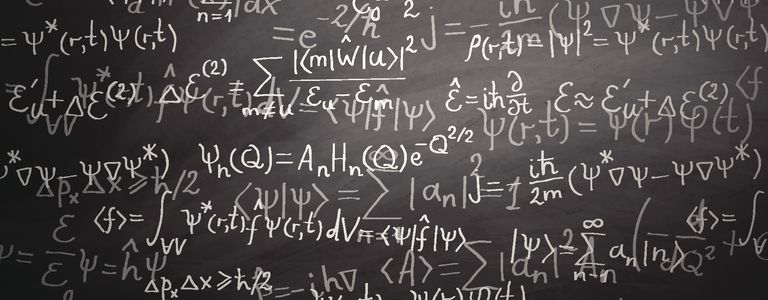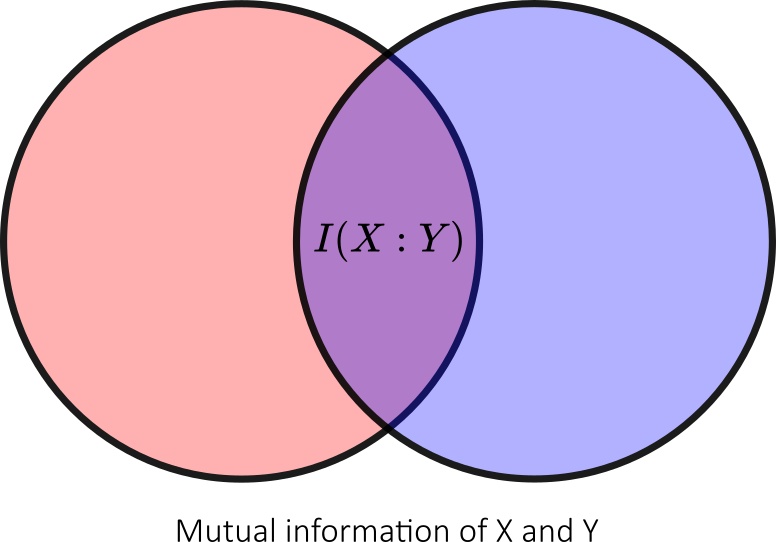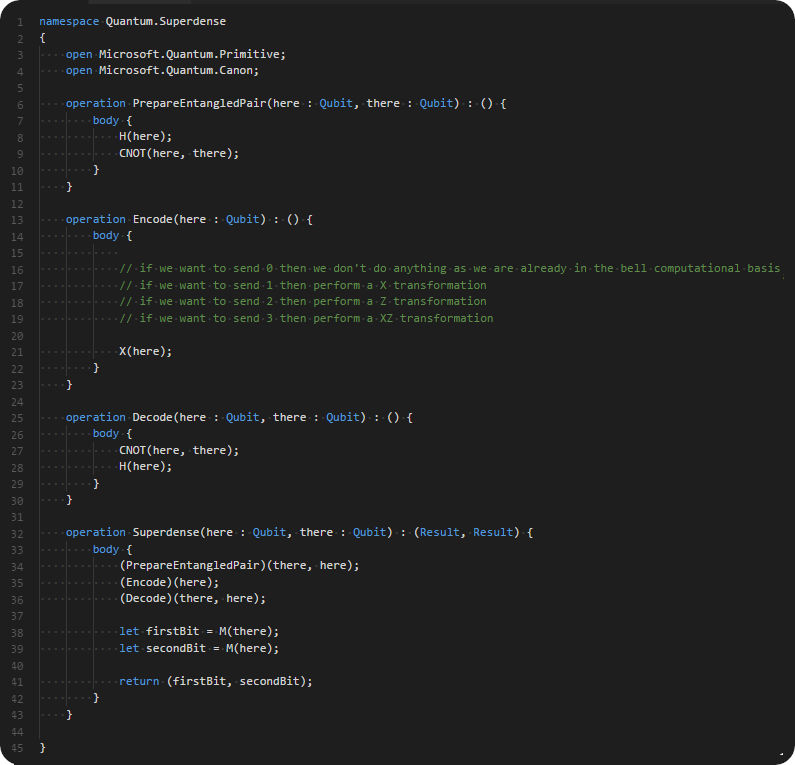# On superdense coding

Alice and Bob are fictional characters often used to describe cryptographic protocols. We introduced them in a previous article on quantum cryptography. Picture a situation where Alice and Bob are living in different parts of the world and where Alice would like to communicate two classical bits of information to Bob by sending him only a single quantum bit.

As usual, simply formulated problems are more complicated than they sound. Superdense coding is a solution to this problem. This is a quantum communication protocol used to transmit two classical bits from Alice to Bob by sending only one qubit, at the condition that Alice and Bob have pre-shared an entangled Bell pair.

It can be thought of as the opposite of quantum teleportation, in which one transfers one qubit from Alice to Bob by communicating two classical bits, as long as Alice and Bob have a pre-shared Bell pair.The HSW theorem and Holevo’s bound

As we guessed earlier, though seemingly simple, the enounced problem turns out to more subtle. The HSW theorem (named after Holevo, Schumacher and Westmoreland) is an important limitative theorem in quantum computing, published in the early 70’s. It is often called the Holevo’s bound, since it establishes an upper bound to the amount of information that can be known about a quantum state.

As in many other fields, in quantum information theory, accessible information is best understood in terms of a 2-party Alice-Bob communication.

Let’s imagine that Alice holds a classical random variable$X$, which can take the values$\{1, 2, \cdots, n\}$ with corresponding probabilities$\{p_1, p_2, \cdots , p_n\}$. Alice then prepares a quantum state, represented by the density matrix$\rho_X$ chosen from a set$\{\rho_1, \rho_2, \cdots, \rho_n\}$, and gives this state to Bob.

Bob’s goal is to find the value of$X$. In order to do that, Bob performs a measurement on the state$\rho_X$, obtaining a classical outcome, which we denote with$Y$.

In this context, the amount of accessible information, that is, the amount of information that Bob can get about the variable$X$, is the maximum value of the mutual information$I(X : Y)$ between the random variables$X$ and$Y$ over all the possible measurements that Bob can do:The mutual information of two random variables$X$ and$Y$ is a measure of the mutual dependence between the two variables. It quantifies the amount of information (bits) obtained about one random variable, through the other random variable. The concept of mutual information is intricately linked to that of entropy of a random variable.

There is currently no known formula to compute the accessible information. There are however several upper bounds, the best-known of which is the Holevo bound, which is specified in the HSW theorem:

•$\displaystyle I(X : Y) \leq S(\rho) - \sum_i p_i S(\rho_i)$
• with$\rho = \sum_i p_i\rho_i$ and where$S$ is the von Neumann entropy.

In essence, the Holevo bound proves that given$n$ qubits, although they can carry a larger amount of classical information (thanks to quantum superposition), the amount of classical information that can be accessed, can be only up to$n$ classical (non-quantum encoded) bits.

This is surprising, for two reasons:

1. that results shows quantum computing to be only as good or inferior to conventional techniques (on that matter);
2. it takes$2^n - 1$ complex numbers to encode the qubits that represent a mere$n$ bits.

Increasing the rate of information with superdense coding

Helovo’s upper bound looks pretty bad for our problem as it is impossible to send two classical bits using one qubit. This would violate HSW’s theorem: sending a single qubit noiselessly between two parties gives a maximum rate of communication of one classical bit per quantum bit.

To get beyond Helovo’s upper bound, one has to pre-share a quantum entanglement before the protocol begins. In these terms, Superdense coding is a quantum communication protocol using a pre-shared quantum entanglement to increase the rate at which information may be sent through a quantum channel.

If the sender’s qubit is maximally entangled (Bell pair) with a qubit in the receiver’s possession, then dense coding increases the maximum rate to two bits per qubit.

Alice and Bob in quantum land

Let’s get an overview of the superdense coding protocol. In the superdense coding scheme, Alice wants to send two classical bits of information (00, 01, 10 or 11) to Bob using qubits (instead of classical bits).

To do that, an entangled Bell state is first prepared. Later on, one of these qubits is sent to Alice and the other to Bob.

Once Alice obtains her entangled qubit, depending on which two-bit message (00, 01, 10 or 11) she wants, a certain quantum gate is applied to her entangled qubit. The entangled qubit is then sent to Bob, who performs a measurement and retrieve the classical two-bit message.

As you have probably noticed, two qubits have to be sent. However, one of these qubits can be provided to Bob in advance, long before the communication, and before the content of the message was decided. Thus, in the instant when you want to send two bits of information, you only have to send one qubit (Alice’s).

This is the idea behind superdense coding,. It illustrates one of the principles of quantum information: you can provide some resource at an earlier time, independent of what is going to be done later and that resource can be consumed to achieve a more efficient result in the instant.

The superdense coding protocol

Let’s get into the details of the superdense coding protocol. The following quantum circuit summarize the necessary steps:1. Step 1 (Preparation): The protocol starts with the preparation of an entangled state. We will use the following quantum circuit we have used many time before:This gate creates a maximally entangled 2-qubit state (Bell pair):$\displaystyle \textsf{CNOT}(H \otimes \mathbf{1}) | 00 \rangle = \frac{1}{\sqrt{2}} \big( | 00 \rangle +| 11 \rangle \big) = | \phi\rangle$

2. Step 2 (Sharing): After the preparation of the Bell pair, a qubit is sent to Alice (it will be denoted by subscript A) and the other qubit is sent to Bob (denoted by subscript B).

Note: at this point, Alice and Bob may be in completely different locations and a long time might elapse between the preparation and sharing of the entangled state and the rest of the steps.

3. Step 3 (Encoding): Alice can transform the shared entangled state$| \phi\rangle$, into any one of the 4 Bell states (including, of course,$| \phi\rangle$). By only manipulating (using quantum gates) her qubit locally, the entanglement between the two qubits will not be broken.

There are 4 cases, which correspond to the 4 possible two-bit strings that Alice may want to send, depending on which classical two-bit message she wants to send to Bob:

 Message (two classical bits) Operation(s) Bell state 00 Identity I$\displaystyle |B_{00}\rangle=\frac{1}{\sqrt{2}} \big( |0_A\rangle\otimes|0_B\rangle + |1_A\rangle\otimes|1_B\rangle \big)$ 01 Pauli bit-flip X$\displaystyle |B_{01}\rangle=\frac{1}{\sqrt{2}} \big( |1_A\rangle\otimes|0_B\rangle + |0_A\rangle\otimes|1_B\rangle \big)$ 10 Pauli phase-flip Z$\displaystyle |B_{10}\rangle=\frac{1}{\sqrt{2}} \big( |0_A\rangle\otimes|0_B\rangle - |0_A\rangle\otimes|0_B\rangle \big)$ 11 Pauli-X followed by Pauli-Z$\displaystyle |B_{11}\rangle=\frac{1}{\sqrt{2}} \big( |0_A\rangle\otimes|1_B\rangle - |1_A\rangle\otimes|0_B\rangle \big)$
4. Step 4 (Sending): After having performed one of the operations above, Alice sends her entangled qubit (of the entangled state shared between her and Bob) to Bob.

5. Step 5 (Decoding): To find which classical bits Alice wanted to send, Bob performs a CNOT operation (with A as control qubit and B as target qubit) and performs a$H \otimes \mathbf{I}$ operation on the entangled qubit A (i.e the Hadamard quantum gate H is only applied to A):These operations are measurements projecting the entangled state onto one of the four 2-qubits basis vectors$| 00 \rangle, | 01 \rangle, | 10 \rangle$ or$|11\rangle$:

1. If the entangled state was$B_{00}$ at step 3, then it becomes$| 00 \rangle$
2. If the entangled state was$B_{01}$ at step 3, then it becomes$| 01 \rangle$
3. If the entangled state was$B_{10}$ at step 3, then it becomes$| 10 \rangle$
4. If the entangled state was$B_{11}$ at step 3, then it becomes$| 11 \rangle$

Security considerations

If an eavesdropper (Eve) intercepts Alice’s qubit en route to Bob, all that is obtained is part of an entangled state. Therefore, no useful information whatsoever is gained by Eve, unless she has also access to Bob’s qubit. Eve cannot tell what information Alice was sending to Bob (the density matrix of that qubit is$\mathbf{I}/2$ no matter what Alice did to it to encode the message).

However, the eavesdropper can scramble the message by applying a Pauli operation on that qubit. Eve won’t know what message Bob will receive (because it will be a combination of what Alice and the eavesdropper did), but then Bob won’t receive what Alice intended.

Please note that there is no authentication of the parties involved whatsoever (for that version of the protocol). Eve could just replace Bob, making the protocol completely insecure.

Superdense coding with IBM’s QISKit

The following python source code (from GitHub) illustrates an implementation of superdense coding using IBM Q > Experience (Terra). Two types of registers (quantum and classical) are needed. The classical registers is used to store the results of measuring our quantum registers. The quantum registers are our qubits. The quantum circuit are used to wire our qubits and operations together:Superdense coding with Microsoft’s Q#

Programming with Microsoft Quantum Development Kit involves two parts. The classical part (using a C#) is used to invoke the quantum simulator, provide input data and read output data from it. The quantum part (using the Q# programming language) creates and uses qubits for algorithms.

The following code excerpt illustrates the steps of the superdense coding protocol:Note: to speedup the writing process, a few paragraphs and illustrations of this post are based on Wikipedia articles on superdense coding and HSW theorem.

This site uses Akismet to reduce spam. Learn how your comment data is processed.